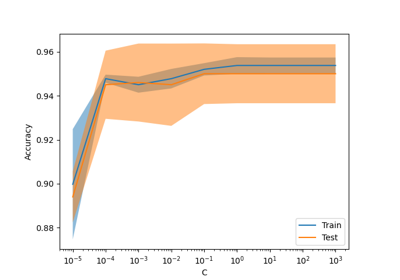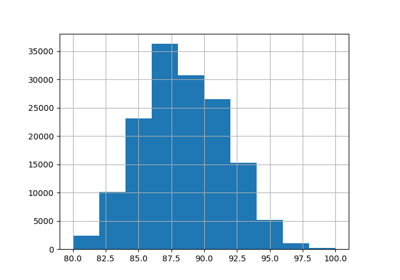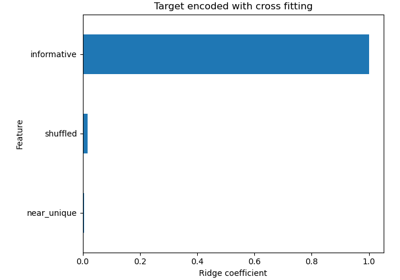# sklearn.preprocessing.TargetEncoder¶

class sklearn.preprocessing.TargetEncoder(categories='auto', target_type='auto', smooth='auto', cv=5, shuffle=True, random_state=None)[source]

Target Encoder for regression and classification targets.

Each category is encoded based on a shrunk estimate of the average target values for observations belonging to the category. The encoding scheme mixes the global target mean with the target mean conditioned on the value of the category. [MIC]

TargetEncoder considers missing values, such as np.nan or None, as another category and encodes them like any other category. Categories that are not seen during fit are encoded with the target mean, i.e. target_mean_.

For a demo on the importance of the TargetEncoder internal cross-fitting, see ref:sphx_glr_auto_examples_preprocessing_plot_target_encoder_cross_val.py. For a comparison of different encoders, refer to Comparing Target Encoder with Other Encoders. Read more in the User Guide.

Note

fit(X, y).transform(X) does not equal fit_transform(X, y) because a cross fitting scheme is used in fit_transform for encoding. See the User Guide for details.

New in version 1.3.

Parameters:
categories“auto” or list of shape (n_features,) of array-like, default=”auto”

Categories (unique values) per feature:

• "auto" : Determine categories automatically from the training data.

• list : categories[i] holds the categories expected in the i-th column. The passed categories should not mix strings and numeric values within a single feature, and should be sorted in case of numeric values.

The used categories are stored in the categories_ fitted attribute.

target_type{“auto”, “continuous”, “binary”}, default=”auto”

Type of target.

• "auto" : Type of target is inferred with type_of_target.

• "continuous" : Continuous target

• "binary" : Binary target

Note

The type of target inferred with "auto" may not be the desired target type used for modeling. For example, if the target consisted of integers between 0 and 100, then type_of_target will infer the target as "multiclass". In this case, setting target_type="continuous" will specify the target as a regression problem. The target_type_ attribute gives the target type used by the encoder.

smooth“auto” or float, default=”auto”

The amount of mixing of the target mean conditioned on the value of the category with the global target mean. A larger smooth value will put more weight on the global target mean. If "auto", then smooth is set to an empirical Bayes estimate.

cvint, default=5

Determines the number of folds in the cross fitting strategy used in fit_transform. For classification targets, StratifiedKFold is used and for continuous targets, KFold is used.

shufflebool, default=True

Whether to shuffle the data in fit_transform before splitting into folds. Note that the samples within each split will not be shuffled.

random_stateint, RandomState instance or None, default=None

When shuffle is True, random_state affects the ordering of the indices, which controls the randomness of each fold. Otherwise, this parameter has no effect. Pass an int for reproducible output across multiple function calls. See Glossary.

Attributes:
encodings_list of shape (n_features,) of ndarray

Encodings learnt on all of X. For feature i, encodings_[i] are the encodings matching the categories listed in categories_[i].

categories_list of shape (n_features,) of ndarray

The categories of each feature determined during fitting or specified in categories (in order of the features in X and corresponding with the output of transform).

target_type_str

Type of target.

target_mean_float

The overall mean of the target. This value is only used in transform to encode categories.

n_features_in_int

Number of features seen during fit.

feature_names_in_ndarray of shape (n_features_in_,)

Names of features seen during fit. Defined only when X has feature names that are all strings.

OrdinalEncoder

Performs an ordinal (integer) encoding of the categorical features. Contrary to TargetEncoder, this encoding is not supervised. Treating the resulting encoding as a numerical features therefore lead arbitrarily ordered values and therefore typically lead to lower predictive performance when used as preprocessing for a classifier or regressor.

OneHotEncoder

Performs a one-hot encoding of categorical features. This unsupervised encoding is better suited for low cardinality categorical variables as it generate one new feature per unique category.

References

Examples

With smooth="auto", the smoothing parameter is set to an empirical Bayes estimate:

>>> import numpy as np
>>> from sklearn.preprocessing import TargetEncoder
>>> X = np.array([["dog"] * 20 + ["cat"] * 30 + ["snake"] * 38], dtype=object).T
>>> y = [90.3] * 5 + [80.1] * 15 + [20.4] * 5 + [20.1] * 25 + [21.2] * 8 +  * 30
>>> enc_auto = TargetEncoder(smooth="auto")
>>> X_trans = enc_auto.fit_transform(X, y)

>>> # A high smooth parameter puts more weight on global mean on the categorical
>>> # encodings:
>>> enc_high_smooth = TargetEncoder(smooth=5000.0).fit(X, y)
>>> enc_high_smooth.target_mean_
44...
>>> enc_high_smooth.encodings_
[array([44..., 44..., 44...])]

>>> # On the other hand, a low smooth parameter puts more weight on target
>>> # conditioned on the value of the categorical:
>>> enc_low_smooth = TargetEncoder(smooth=1.0).fit(X, y)
>>> enc_low_smooth.encodings_
[array([20..., 80..., 43...])]


Methods

 fit(X, y) Fit the TargetEncoder to X and y. fit_transform(X, y) Fit TargetEncoder and transform X with the target encoding. get_feature_names_out([input_features]) Get output feature names for transformation. Get metadata routing of this object. get_params([deep]) Get parameters for this estimator. set_output(*[, transform]) Set output container. set_params(**params) Set the parameters of this estimator. Transform X with the target encoding.
fit(X, y)[source]

Fit the TargetEncoder to X and y.

Parameters:
Xarray-like of shape (n_samples, n_features)

The data to determine the categories of each feature.

yarray-like of shape (n_samples,)

The target data used to encode the categories.

Returns:
selfobject

Fitted encoder.

fit_transform(X, y)[source]

Fit TargetEncoder and transform X with the target encoding.

Note

fit(X, y).transform(X) does not equal fit_transform(X, y) because a cross fitting scheme is used in fit_transform for encoding. See the User Guide. for details.

Parameters:
Xarray-like of shape (n_samples, n_features)

The data to determine the categories of each feature.

yarray-like of shape (n_samples,)

The target data used to encode the categories.

Returns:
X_transndarray of shape (n_samples, n_features)

Transformed input.

get_feature_names_out(input_features=None)[source]

Get output feature names for transformation.

Parameters:
input_featuresarray-like of str or None, default=None

Input features.

• If input_features is None, then feature_names_in_ is used as feature names in. If feature_names_in_ is not defined, then the following input feature names are generated: ["x0", "x1", ..., "x(n_features_in_ - 1)"].

• If input_features is an array-like, then input_features must match feature_names_in_ if feature_names_in_ is defined.

Returns:
feature_names_outndarray of str objects

Same as input features.

Get metadata routing of this object.

Please check User Guide on how the routing mechanism works.

Returns:

A MetadataRequest encapsulating routing information.

get_params(deep=True)[source]

Get parameters for this estimator.

Parameters:
deepbool, default=True

If True, will return the parameters for this estimator and contained subobjects that are estimators.

Returns:
paramsdict

Parameter names mapped to their values.

property infrequent_categories_

Infrequent categories for each feature.

set_output(*, transform=None)[source]

Set output container.

See Introducing the set_output API for an example on how to use the API.

Parameters:
transform{“default”, “pandas”}, default=None

Configure output of transform and fit_transform.

• "default": Default output format of a transformer

• "pandas": DataFrame output

• None: Transform configuration is unchanged

Returns:
selfestimator instance

Estimator instance.

set_params(**params)[source]

Set the parameters of this estimator.

The method works on simple estimators as well as on nested objects (such as Pipeline). The latter have parameters of the form <component>__<parameter> so that it’s possible to update each component of a nested object.

Parameters:
**paramsdict

Estimator parameters.

Returns:
selfestimator instance

Estimator instance.

transform(X)[source]

Transform X with the target encoding.

Note

fit(X, y).transform(X) does not equal fit_transform(X, y) because a cross fitting scheme is used in fit_transform for encoding. See the User Guide. for details.

Parameters:
Xarray-like of shape (n_samples, n_features)

The data to determine the categories of each feature.

Returns:
X_transndarray of shape (n_samples, n_features)

Transformed input.

## Examples using sklearn.preprocessing.TargetEncoder¶Release Highlights for scikit-learn 1.3

Release Highlights for scikit-learn 1.3Comparing Target Encoder with Other Encoders

Comparing Target Encoder with Other EncodersTarget Encoder’s Internal Cross fitting

Target Encoder's Internal Cross fitting